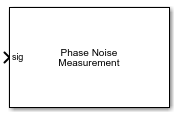# Phase Noise Measurement

Compute phase noise at specific frequency offset vectors

• Library:
• Mixed-Signal Blockset / PLL / Measurements & Testbenches## Description

The Phase Noise Measurement block measures the phase noise at the specific frequency offset vectors at the output of a voltage controlled oscillator (VCO) or a phase-locked loop (PLL).

## Ports

### Input

expand all

Input clock signal to the Phase Noise Measurement block, specified as a scalar. The sig port is connected to the output of a VCO.

Data Types: `double`

## Parameters

expand all

Sample rate of the input signal, specified as a real positive scalar in Hz. Sampling frequency is used to configure the Spectrum Estimator inside the VCO block. To achieve the best performance metrics, specify a sample rate at least eight times the operating frequency of the VCO.

#### Programmatic Use

• Use `get_param(gcb,'SampleRate')` to view the current value of Sampling frequency.

• Use `set_param(gcb,'SampleRate',value)` to set Sampling frequency to a specific value.

Resolution bandwidth of the Spectrum Estimator inside the PLL DUT, specified as a real positive scalar. The Spectrum Estimator inside the VCO uses the Resolution bandwidth to determine window length for spectral analysis using the Welch method.

In general, Resolution bandwidth should be less than or equal to the lowest offset frequency from the phase noise data.

#### Programmatic Use

• Use `get_param(gcb,'ResBandwidth')` to view the current value of Resolution bandwidth.

• Use `set_param(gcb,'ResBandwidth',value)` to set Resolution bandwidth to a specific value.

Number of spectral averages, specified as a positive integer scalar. The Spectrum Estimator inside the VCO computes the current power spectrum estimate by averaging over the number specified by No. of spectral averages.

#### Programmatic Use

• Use `get_param(gcb,'SpectralAverages')` to view the current value of No. of spectral averages.

• Use `set_param(gcb,'SpectralAverages',value)` to set No. of spectral averages to a specific value.

Delays measurement analysis by the specified amount of time to avoid corruption by transients, specified as a real nonnegative scalar in s.

#### Programmatic Use

• Use `get_param(gcb,'HoldOffTime')` to view the current value of Hold off time.

• Use `set_param(gcb,'HoldOffTime',value)` to set Hold off time to a specific value.

Frequency points relative to fundamental frequency where phase noise is calculated, specified as a real valued vector in Hz.

#### Programmatic Use

• Use `get_param(gcb,'PhaseNoiseFreqOffset')` to view the current value of Frequency offset vector.

• Use `set_param(gcb,'PhaseNoiseFreqOffset',value)` to set Frequency offset vector to a specific value.

Select to enable increased buffer size during simulation. This increases the buffer size of all the blocks in the PLL model that belong to the Mixed-Signal Blockset™/PLL/Building Blocks library. By default, this option is deselected.

Number of samples of the input buffering available during simulation, specified as a positive integer scalar. This sets the buffer size of all the blocks in the PLL model that belong to the Mixed-Signal Blockset/PLL/Building Blocks library.

Selecting different simulation solver or sampling strategies can change the number of input samples needed to produce an accurate output sample. Set the Buffer size to a large enough value so that the input buffer contains all the input samples required.

#### Dependencies

This parameter is only available when Enable increased buffer size parameter is selected.

#### Programmatic Use

• Use `get_param(gcb,'TargetPhaseNoiseVector')` to view the current value of Phase noise.

• Use `set_param(gcb,'TargetPhaseNoiseVector',value)` to set Phase noise to a specific value.

Click to plot the phase noise profile of the PLL device (DUT) and to compare it with the user-defined phase noise profile after simulation is complete.

Click to export measurement results to an excel spreadsheet (XLS) or as comma-separated values (CSV) after the simulation is complete.

#### Introduced in R2019a

##### SupportGet trial now